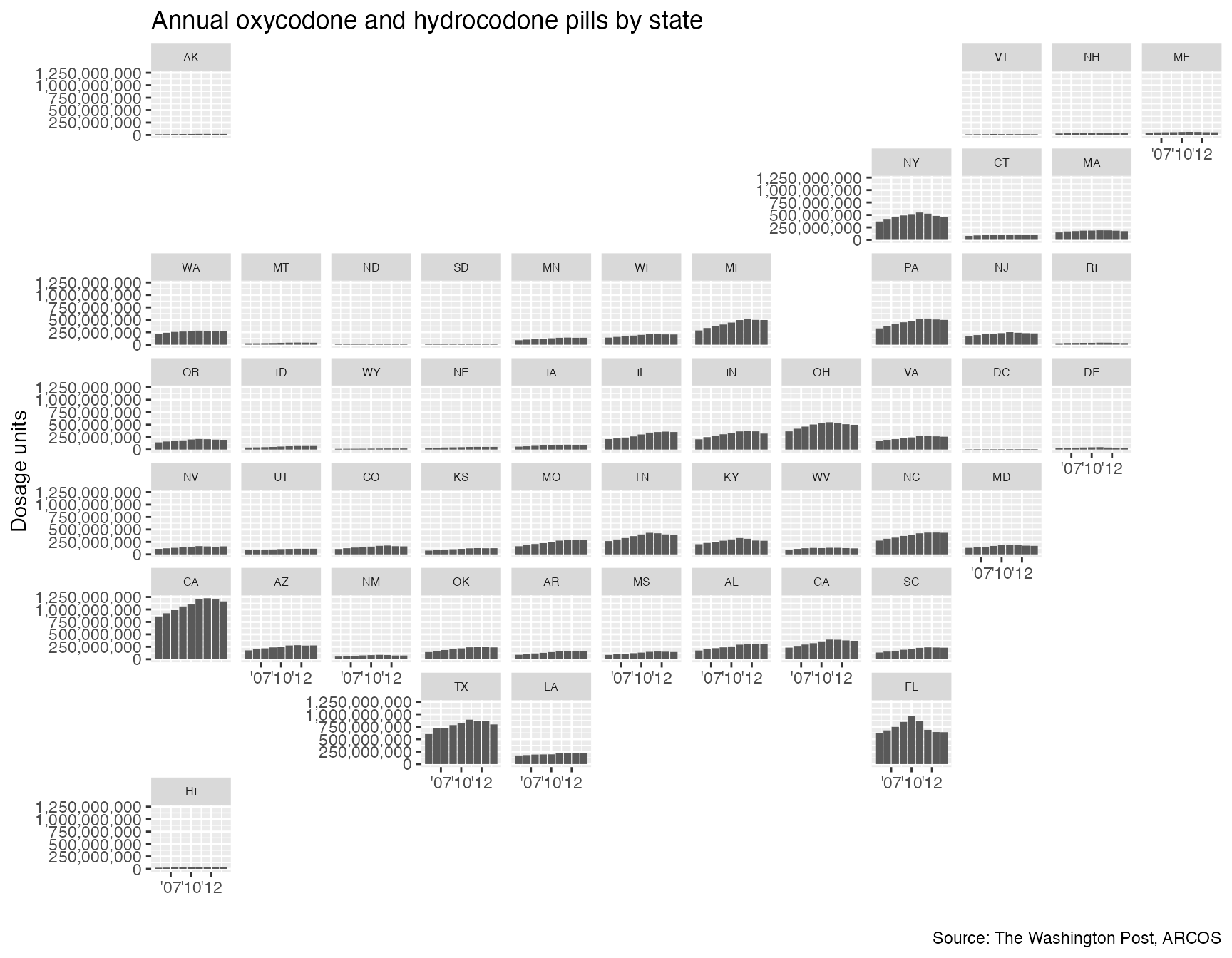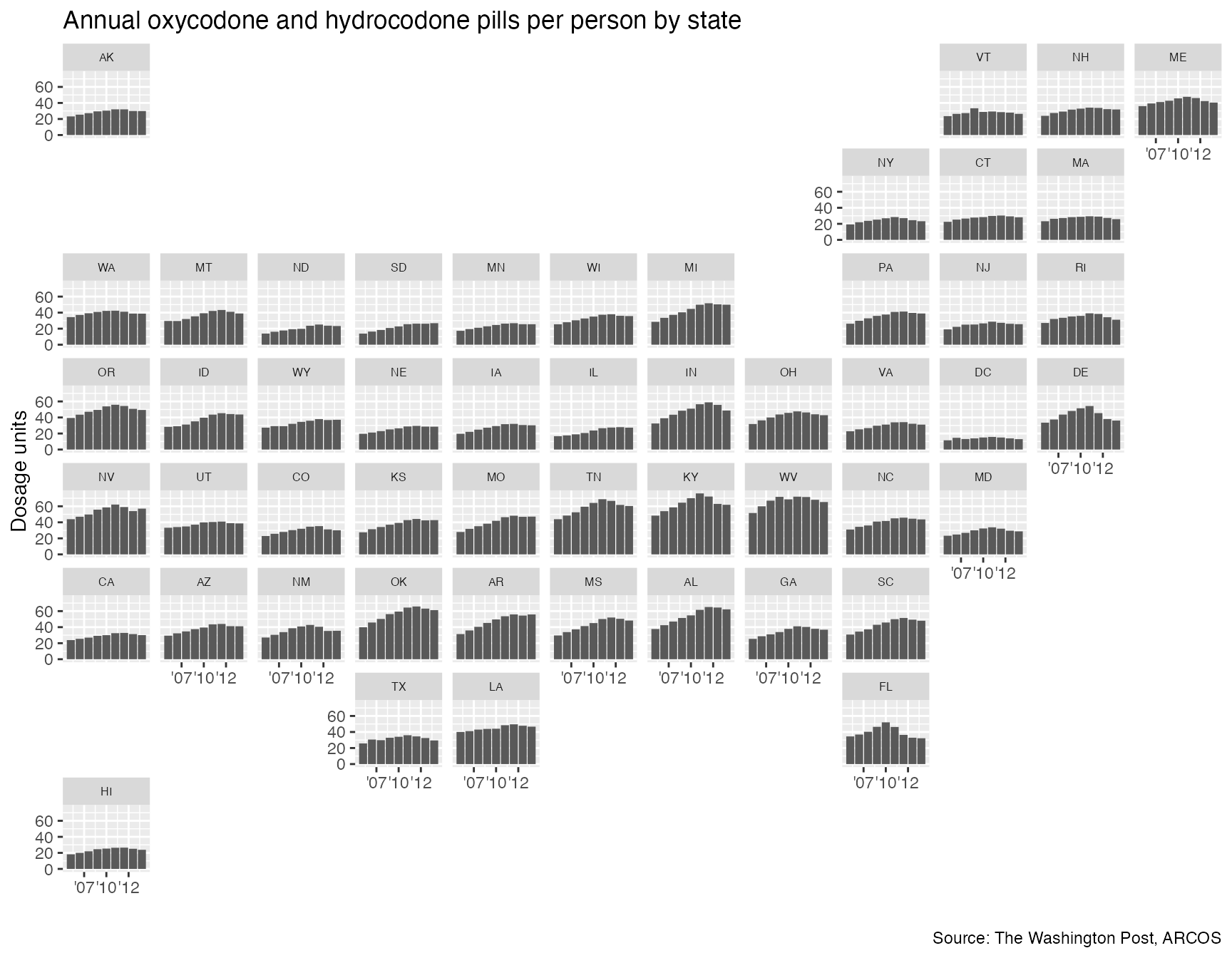How to make some quick maps with the ARCOS api.

# Uncomment and run the lines below to see if you have the packages required already installed
# packages <- c("dplyr", "ggplot2", "jsonlite", "knitr", "geofacet", "scales")
# if (length(setdiff(packages, rownames(installed.packages()))) > 0) {
#   install.packages(setdiff(packages, rownames(installed.packages())), repos = "http://cran.us.r-project.org") # }

# These are all the packages you'll need to run everything below

library(dplyr)
library(ggplot2)
library(arcos)
library(jsonlite)
library(knitr)
library(geofacet)
library(scales)
states <- combined_buyer_annual(key="WaPo")

kable(head(states))
A90777889 RETAIL PHARMACY KINGS NY 2006 110700
A90777889 RETAIL PHARMACY KINGS NY 2007 119100
A90777889 RETAIL PHARMACY KINGS NY 2008 100300
A90777889 RETAIL PHARMACY KINGS NY 2009 92630
A90777889 RETAIL PHARMACY KINGS NY 2010 65860
A90777889 RETAIL PHARMACY KINGS NY 2011 72030

We’ve pulled every pharmacy across the country and how many pills they sold per year.

Let’s aggregate and combine by states and year.

annual_states <- states %>%
summarize(pills=sum(DOSAGE_UNIT)) %>%
#> summarise() regrouping output by 'BUYER_STATE' (override with .groups argument)

kable(head(annual_states))
AE 2006 330
AK 2006 15667010
AK 2007 17272433
AK 2008 18707811
AK 2009 20160949
AK 2010 21012703

Looks good. Let’s use the geofacet package to map out the annual pill trends.

ggplot(annual_states, aes(year, pills)) +
geom_col() +
facet_geo(~ BUYER_STATE, grid = "us_state_grid2") +
scale_x_continuous(labels = function(x) paste0("'", substr(x, 3, 4))) +
scale_y_continuous(label=comma) +
labs(title = "Annual oxycodone and hydrocodone pills by state",
caption = "Source: The Washington Post, ARCOS",
x = "",
y = "Dosage units") +
theme(strip.text.x = element_text(size = 6))This is nice but Florida, California, and Texas stand out because of their higher population.

We should normalize this with population.

Get the annual state population with state_population().

population <- state_population(key="WaPo")

kable(head(population))
AL 2009 4633360
AK 2009 683142
AZ 2009 6324865
AR 2009 2838143
CA 2009 36308527
CO 2009 4843211

Now, we join the two data sets.

annual_states_joined <- left_join(annual_states, population) %>%
filter(!is.na(population))
#> Joining, by = c("BUYER_STATE", "year")

kable(head(annual_states_joined))
AK 2006 15667010 675302
AK 2007 17272433 680300
AK 2008 18707811 687455
AK 2009 20160949 683142
AK 2010 21012703 691189
AK 2011 22444775 700703

Do some math…

annual_states_joined <- annual_states_joined %>%
mutate(pills_per=pills/population)

kable(head(annual_states_joined))
AK 2006 15667010 675302 23.20001
AK 2007 17272433 680300 25.38944
AK 2008 18707811 687455 27.21314
AK 2009 20160949 683142 29.51209
AK 2010 21012703 691189 30.40081
AK 2011 22444775 700703 32.03180

Map it out again this time per capita.


ggplot(annual_states_joined, aes(year, pills_per)) +
geom_col() +
facet_geo(~ BUYER_STATE, grid = "us_state_grid2") +
scale_x_continuous(labels = function(x) paste0("'", substr(x, 3, 4))) +
scale_y_continuous(label=comma) +
labs(title = "Annual oxycodone and hydrocodone pills per person by state",
caption = "Source: The Washington Post, ARCOS",
x = "",
y = "Dosage units") +
theme(strip.text.x = element_text(size = 6))